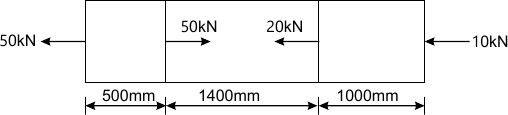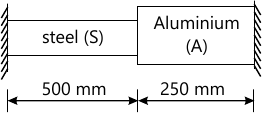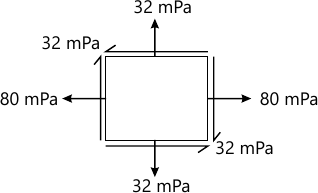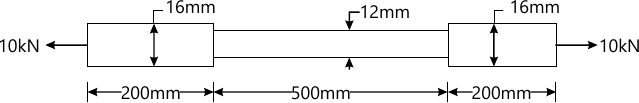MORE IN Mechanics of Materials
VTU Mechanical Engineering (Semester 3)
Mechanics of Materials
June 2013
Total marks: --
Total time: --
INSTRUCTIONS
(1) Assume appropriate data and state your reasons
(2) Marks are given to the right of every question
(3) Draw neat diagrams wherever necessary

1 (a) The tensile test was conducted on a mild steel bar .The following data was obtained from the test:
Diameter of steel bar =16mm
Gauge lenghth of the bar =80mm
Extension at a load of 60kN=0.115mm
final gauge lenghth of bar =104mm
Diameter of the load at failure=12mm
determine
young's module;proportionalty limit; true breaking stress and ;percentage elogation.
10 M
1 (b) A brass hving cross -sectional area 300mm3is subjected to axial forces as shown in fig Q.1(b).find the total elongation of the bar.E=84GPa.10 M

2 (a) A bar of 20mm diameter is tested in tension.it is observed that when a laod of 37.7kN is applied,the extension measured over length of 200mm is 1.12mm and contraction in diameter is 0.0036mm.Find possion's ration elastic constant E,G,k.
8 M
2 (b) A composite bar made up of aluminium ans steel at a temoreture of 42°C.What will be the stresses in the two bar with the tempreture drops to 24°C.If
The supports are unyielding ;
the support come nearer to each other by 0.1mm.The cross-sectional area of steel bar is 160mm2.Ea=0.7×105MPa,Es=2×105MPa,?=24×10-6 par °C and &props=12×10-6,per °C12 M

3 (a) Show that the sum of the normal streses on any two planes at right angles in a general two dimensional stress system is constant.
8 M
3 (b) At a certain point in strained material the value of normals streses across two planes at right angles to each other are 80MPa and 32MPz. both tensile and there is shear stress of 32MPa clock wise on the palnes carrrying 80Pa sresses across the planes as shown in fig.Q.3(b). Dtermine:
Maximum and mInimum normal stresses and locate their planes
Maximum shear stress and specify its plane12 M

4 (a) State castigliano's theorem.where do you use it?
3 M
4 (b) The bar with circular cross -section as shown in fig.Q.(b) Is subjected to a load of 10kN.
Determine the strain energy stored in it .Take E=2.1×105n/mm210 M
4 (c) A thin cylindrical shell 1 m in diameter and 3m long has a metal thickness of 10mm.It subjected to an internal fluid pressure of 3MPa.Determine the chenge in lenghth,diamet and volume .Also find the maximum shaering stress in the shell.Assume poison ratio 0.3 and E=210GPa.
10 M

5 (a) Expalin the terms:
Sagging bending moment
Hogging bending moment
Point of contraflexture
6 M
5 (b) What are the different types of load acting on a beam?Explain with sketches
6 M
5 (c) A simply supported beam of span 6m is subjected to a concentrated load of 25kN acting distance of 2m from the left end .Also subjected to an uniformaly distributed load of 10kN/m over the entire span .draw the bending moment and shear force diagrams indicating the maximum and minimum values
8 M

6 (a) Enumerate the assumption made in the theory of simple bending
4 M
6 (b) A cantilever of square section 200mm×200mm,2m long .just fails in flexture when of 12kN is placed at is free end.A beam of the same material and having a rectangular cross section 150mm wide and 300mm deep is simply supported over a span of 3m.Calculate the minimum central concentrated load required to break the beam
8 M
6 (C) A rolled I section of size 50mm×75mm is used as a beam,with an effective spam 3 meters.The flangers are 5mm thick and web 3.75mm thik.calculate the uniformaly distributed load the beam can carry if the maximum intensity of stress induced limited to 40N/mm2
8 M

7 (a) Show that for a simply supported beam of length l carrying a concentrated load W at its mid span ,the maximumdefection in W/48EL
10 M
7 (b) A simply supported steel beam having uniform cross is 14m spam and is simply supported at its ends .It carries a concentrated load of 120kN and 80kN at two points 3m and 4.5m from the left and right end respectivel.if the moment of inertia of the section is 160×102mm and E= 210GPa.calculate the deflection of the beam at the load points
10 M

8 (a) a hollow circular steel shaft has to tranmit 60k W at 210rpm such that the maximum shear stress does not exceed 60mpa.If the ration of internal to external diameter is equal to 3&fraction;4 and the value of rigidity modulus is 84 Gpa.find the diamension of the shaff and angel of twist in alength of 3m
10 M
8 (b) A 1.5m long column has a circular cross section of 50mm diameter.One end of the column is fixed in direction and position and the other end is free.taking the factor of safety as 3.calculate the safe load using.
Rankine's formula taking yield stress 560N/mm2 and ?=1&fraction;1600.
Euler's formula taking E=1.2&fraction;105N/mm2.
10 M

More question papers from Mechanics of Materials